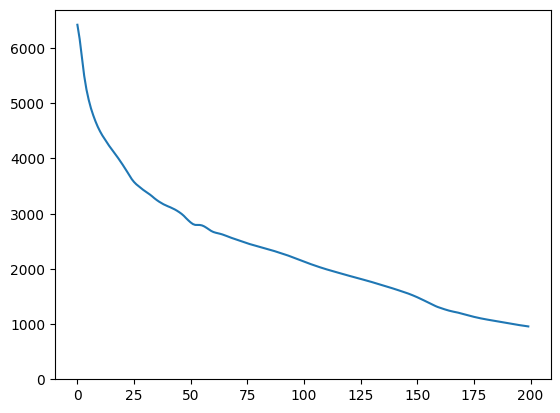# 使用 CelebA 渐进式 GAN 模型生成人工面部

• 从隐空间映射到图像，以及
• 提供一个目标图像，利用梯度下降法找到生成与目标图像相似的图像的隐向量。

## 设置

````# Install imageio for creating animations.`
`pip -q install imageio`
`pip -q install scikit-image`
`pip install git+https://github.com/tensorflow/docs`
```

### Imports and function definitions

``````from absl import logging

import imageio
import PIL.Image
import matplotlib.pyplot as plt
import numpy as np

import tensorflow as tf
tf.random.set_seed(0)

import tensorflow_hub as hub
from tensorflow_docs.vis import embed
import time

try:
except ImportError:
pass

from IPython import display
from skimage import transform

# We could retrieve this value from module.get_input_shapes() if we didn't know
# beforehand which module we will be using.
latent_dim = 512

# Interpolates between two vectors that are non-zero and don't both lie on a
# line going through origin. First normalizes v2 to have the same norm as v1.
# Then interpolates between the two vectors on the hypersphere.
def interpolate_hypersphere(v1, v2, num_steps):
v1_norm = tf.norm(v1)
v2_norm = tf.norm(v2)
v2_normalized = v2 * (v1_norm / v2_norm)

vectors = []
for step in range(num_steps):
interpolated = v1 + (v2_normalized - v1) * step / (num_steps - 1)
interpolated_norm = tf.norm(interpolated)
interpolated_normalized = interpolated * (v1_norm / interpolated_norm)
vectors.append(interpolated_normalized)
return tf.stack(vectors)

# Simple way to display an image.
def display_image(image):
image = tf.constant(image)
image = tf.image.convert_image_dtype(image, tf.uint8)
return PIL.Image.fromarray(image.numpy())

# Given a set of images, show an animation.
def animate(images):
images = np.array(images)
converted_images = np.clip(images * 255, 0, 255).astype(np.uint8)
imageio.mimsave('./animation.gif', converted_images)
return embed.embed_file('./animation.gif')

logging.set_verbosity(logging.ERROR)
``````

## 隐空间插值法

### 随机向量

``````progan = hub.load("https://tfhub.dev/google/progan-128/1").signatures['default']
``````
```2021-08-13 20:59:43.983216: I tensorflow/stream_executor/cuda/cuda_gpu_executor.cc:937] successful NUMA node read from SysFS had negative value (-1), but there must be at least one NUMA node, so returning NUMA node zero
2021-08-13 20:59:43.991339: I tensorflow/stream_executor/cuda/cuda_gpu_executor.cc:937] successful NUMA node read from SysFS had negative value (-1), but there must be at least one NUMA node, so returning NUMA node zero
2021-08-13 20:59:43.992306: I tensorflow/stream_executor/cuda/cuda_gpu_executor.cc:937] successful NUMA node read from SysFS had negative value (-1), but there must be at least one NUMA node, so returning NUMA node zero
2021-08-13 20:59:43.993902: I tensorflow/core/platform/cpu_feature_guard.cc:142] This TensorFlow binary is optimized with oneAPI Deep Neural Network Library (oneDNN) to use the following CPU instructions in performance-critical operations:  AVX2 AVX512F FMA
To enable them in other operations, rebuild TensorFlow with the appropriate compiler flags.
2021-08-13 20:59:43.994550: I tensorflow/stream_executor/cuda/cuda_gpu_executor.cc:937] successful NUMA node read from SysFS had negative value (-1), but there must be at least one NUMA node, so returning NUMA node zero
2021-08-13 20:59:43.995583: I tensorflow/stream_executor/cuda/cuda_gpu_executor.cc:937] successful NUMA node read from SysFS had negative value (-1), but there must be at least one NUMA node, so returning NUMA node zero
2021-08-13 20:59:43.996481: I tensorflow/stream_executor/cuda/cuda_gpu_executor.cc:937] successful NUMA node read from SysFS had negative value (-1), but there must be at least one NUMA node, so returning NUMA node zero
2021-08-13 20:59:44.599599: I tensorflow/stream_executor/cuda/cuda_gpu_executor.cc:937] successful NUMA node read from SysFS had negative value (-1), but there must be at least one NUMA node, so returning NUMA node zero
2021-08-13 20:59:44.600655: I tensorflow/stream_executor/cuda/cuda_gpu_executor.cc:937] successful NUMA node read from SysFS had negative value (-1), but there must be at least one NUMA node, so returning NUMA node zero
2021-08-13 20:59:44.601636: I tensorflow/stream_executor/cuda/cuda_gpu_executor.cc:937] successful NUMA node read from SysFS had negative value (-1), but there must be at least one NUMA node, so returning NUMA node zero
2021-08-13 20:59:44.602622: I tensorflow/core/common_runtime/gpu/gpu_device.cc:1510] Created device /job:localhost/replica:0/task:0/device:GPU:0 with 14648 MB memory:  -> device: 0, name: Tesla V100-SXM2-16GB, pci bus id: 0000:00:05.0, compute capability: 7.0
2021-08-13 20:59:45.021380: I tensorflow/compiler/mlir/mlir_graph_optimization_pass.cc:185] None of the MLIR Optimization Passes are enabled (registered 2)
```
``````def interpolate_between_vectors():
v1 = tf.random.normal([latent_dim])
v2 = tf.random.normal([latent_dim])

# Creates a tensor with 25 steps of interpolation between v1 and v2.
vectors = interpolate_hypersphere(v1, v2, 50)

# Uses module to generate images from the latent space.
interpolated_images = progan(vectors)['default']

return interpolated_images

interpolated_images = interpolate_between_vectors()
animate(interpolated_images)
``````
```2021-08-13 20:59:45.887048: I tensorflow/stream_executor/cuda/cuda_dnn.cc:369] Loaded cuDNN version 8100
2021-08-13 20:59:46.444959: I tensorflow/core/platform/default/subprocess.cc:304] Start cannot spawn child process: No such file or directory
```## 查找隐空间中的最近向量

``````image_from_module_space = True  # @param { isTemplate:true, type:"boolean" }

def get_module_space_image():
vector = tf.random.normal([1, latent_dim])
images = progan(vector)['default']
return images

return transform.resize(image, [128, 128])

if image_from_module_space:
target_image = get_module_space_image()
else:

display_image(target_image)
````````````tf.random.set_seed(42)
initial_vector = tf.random.normal([1, latent_dim])
``````
``````display_image(progan(initial_vector)['default'])
````````````def find_closest_latent_vector(initial_vector, num_optimization_steps,
steps_per_image):
images = []
losses = []

vector = tf.Variable(initial_vector)
loss_fn = tf.losses.MeanAbsoluteError(reduction="sum")

for step in range(num_optimization_steps):
if (step % 100)==0:
print()
print('.', end='')
if (step % steps_per_image) == 0:
images.append(image.numpy())
target_image_difference = loss_fn(image, target_image[:,:,:3])
# The latent vectors were sampled from a normal distribution. We can get
# more realistic images if we regularize the length of the latent vector to
# the average length of vector from this distribution.
regularizer = tf.abs(tf.norm(vector) - np.sqrt(latent_dim))

loss = target_image_difference + regularizer
losses.append(loss.numpy())

return images, losses

num_optimization_steps=200
steps_per_image=5
images, loss = find_closest_latent_vector(initial_vector, num_optimization_steps, steps_per_image)
``````
```....................................................................................................
....................................................................................................
```
``````plt.plot(loss)
plt.ylim([0,max(plt.ylim())])
``````
```(0.0, 6696.268389892578)
`````````animate(np.stack(images))
````````````display_image(np.concatenate([images[-1], target_image], axis=1))
``````### 试运行上述示例

• 对图像差异使用不同的损失，例如二次方程，
• 对隐向量使用不同的正则化器，
• 在多次运行中从随机向量初始化，
• 等等。
[{ "type": "thumb-down", "id": "missingTheInformationINeed", "label":"没有我需要的信息" },{ "type": "thumb-down", "id": "tooComplicatedTooManySteps", "label":"太复杂/步骤太多" },{ "type": "thumb-down", "id": "outOfDate", "label":"内容需要更新" },{ "type": "thumb-down", "id": "translationIssue", "label":"翻译问题" },{ "type": "thumb-down", "id": "samplesCodeIssue", "label":"Samples / code issue" },{ "type": "thumb-down", "id": "otherDown", "label":"其他" }]
[{ "type": "thumb-up", "id": "easyToUnderstand", "label":"易于理解" },{ "type": "thumb-up", "id": "solvedMyProblem", "label":"解决了我的问题" },{ "type": "thumb-up", "id": "otherUp", "label":"其他" }]